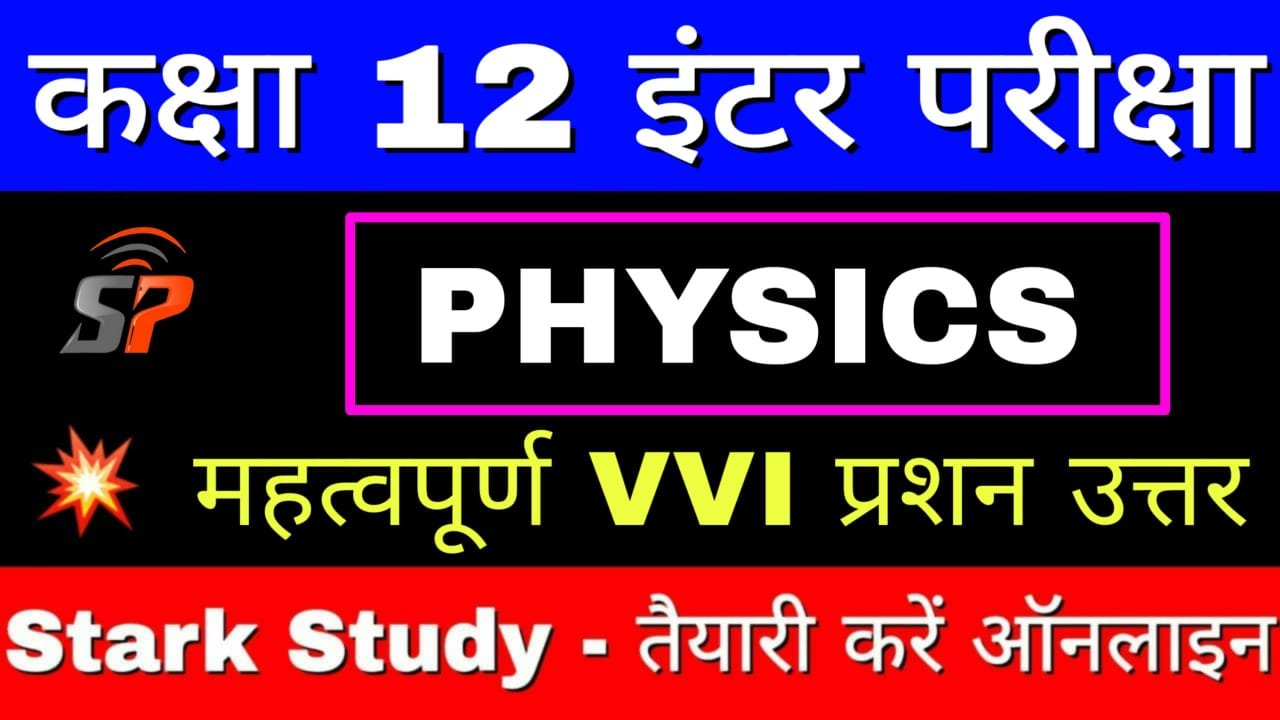BSEB 12th Physics Objective Question Paper Dual Nature Of Matter And Radiation Chapter Objective

DUAL NATURE OF MATTER AND RADIATION

1. Which one of the following is deflected in electric field ?

(A) Gamma rays

(B) X-rays.

(C) Ultraviolet rays

(D) Cathode rays

2. Wavelength of a particles of mass m moving with velocity V is :

(A) λ = mV / h

(B) λ = h / mV

(C) λ = h. mV

(D) λ = mV / h2

3. Who established that electric charge is quantised ?

(A) J.J. Thomson

(b) William Crookes

(C) R.A. Millikan

(D) Wilhelm Rontgen

4. Cathode rays were discovered by :

(A) Maxwell Clerk James

(B) Heinrich Hertz

(C) William Crookes

(D) J. J. Thomson

5. Definite value of energy possessed by a quantum of radiation is called :

(A) Proton

(B) Photon

(C) Deuteron

(D) Lepton

6. The phenomenon of photoelectric emission was discovered in 1887 by :

(A) Albert Einstein

(B) Heinrich Hertz

(c) Wilhelm Hallwachs

(D) Philipp Lenard

7. Which one among the following shows particle nature of light?

(A) Photoelectric effect

(B) Interference

(C) Refraction

(D) Polarization

8. The maximum value of photoelectric current is called :

(A) base current

(B) saturation current

(C) collector current

(D) emitter current

9. The time taken by a photoelectron to come out after the photon strikes is approximately :

(A) 10-1s

(B) 10-4 s

(C) 10-10

(D) 10-16

10. Who won the Nobel Prize in physics in the year 1929 for the discovery of the wave nature of electrons ?

(A) Erwin Schrodinger

(B) R.A. Millikan

(C) Louis Victor de Broglie

(D) Albert Einstein

11. The rest mass of photon is :

(A) hν / c

(B) hν / c2

(C) hν / λ

(D) zero

12. When the velocity of an electron increases, its de Broglie wavelength :

(A) increases

(B) decreases

(C) remains same

(D) may increase or decrease

13. Which of the following devices is sometimes called an electric eye?

(A) LED

(B) Photocell

(C) Integrated chip (IC)

(D) Solar cell

14. The de Broglie wavelength is given by :

(A) P = 2πh / λ

(B) P = h / 2λ

(C) P = 2π / hλ

(D) P= 2π / λ

15. Which of these particles having the same kinetic energy has the largest de Broglie wavelength ?

(A) Electron

(B) Alpha particle

(C) Proton

(D) Neutron

16. X-rays are :

(A) deflected by an electric field

(B) deflected by a magnetic field

(C) deflected by both electric and magnetic fields

(D) not deflected by electric and magnetic fields

17. Which of the following radiations cannot eject photo electrons ?

(A) ultraviolet

(B) infrared

(C) visible

(D) X-rays

18. Evidence of the wave nature of light cannot be obtained from:

(A) diffraction

(B) interference

(C) doppler effect”

(D) reflection

19. The charge of a photo electron is :

(A) 9.1 x 10-31 C

(B) 9.1 x 10-27 C

(C) 9.1 x 10-24 C

(D) none of these

20. A photocell converts light energy into :

(A) electrical energy

(B) magnetic energy

(C) sound energy

(D) None of these

21. In which of the following phenomenon does light intera with matter ?

(A) diffraction

(B) polarisation

(C) dispersion

(D) photoelectric effect

22. Photoelectric effect was discovered by :

(A) Hertz

(B) Hallwaqchs

(C) Lenard

(D) MKillikan

23. Photoelectric effect can be caused by :

(A) X-rays

(B) Visible light

(C) γ-rays

(D) All of these

24. β-rays are deflected in:

(A) gravitational field

(B) only in magnetic field

(C) only in electric field

(D) both in magnetic and electric fields

25. The energy of a photon of wavelength λ is:

(A) hcλ

(B) hc / λ

(C) hλ / c

(D) λ / hc

26. When light enters into glass its Wavelength :

(A) Decreases

(B) Increases

(C) Remains the same

(D) None of these

27. Dimention of plank constant is :

(A) MLT-1

(B) MLT-2

(C) MLT-1

(D) MLT2

28. The energy of emitted photo electron depends upon :

(A) Intensity of light

(B) Wave length of light

(C) Work-function of metal

(D) None of these

29. The critical angle of light passing from glass to air is minimum for:

(A) Red colours

(B) Green colour

(C) Yellow colour

(D) Violet colour

30. Transverse nature of light is shown by :

(A) Interference

(B) Reflection

(C) Polarisation

(D) Dispersion

31. The minimum angular momentum of electron in Hydrogen atom will be:

(A) h / π Js

(B) h / 2π Js

(C) hπ Js

(D) 2π h Js

32. Kirchhoffe’s second law of conservation of :-

(A) charge

(B) energy

(C) momentum

(D) angular momentum

33. Life of a fossil in estimated by :

(A) carbon dating

(B) X-ray

(C) y-ray

(D) laser